# Wigner D-matrix

The Wigner D-matrix is a matrix in an irreducible representation of the groups SU(2) and SO(3). The complex conjugate of the elements of the D-matrix with integral indices are eigenfunctions of the Hamiltonian of spherical and symmetric rigid rotors. The matrix was introduced by E. Wigner in 1927

## Contents

###  Definition Wigner D-matrix

Let jx, jy, jz be generators of the Lie algebra of SU(2) and SO(3). In quantum mechanics these three operators are the components of a vector operator known as angular momentum. Examples are the angular momentum of an electron in an atom, electronic spin,and the angular momentum of a rigid rotor. In all cases the three operators satisfy the following commutation relations,$[j_x,j_y] = i j_z,\quad [j_z,j_x] = i j_y,\quad [j_y,j_z] = i j_x,$

where i is the purely imaginary number and Planck's constant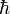$\hbar$ has been put equal to one. The operator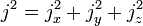$j^2 = j_x^2 + j_y^2 + j_z^2$

is a Casimir operator of SU(2) (or SO(3) as the case may be). It may be diagonalized together with jz (the choice of this operator is a convention), which commutes with j2. That is, it can be shown that there is a complete set of kets with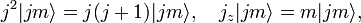$j^2 |jm\rangle = j(j+1) |jm\rangle,\quad j_z |jm\rangle = m |jm\rangle,$

where$j=0, \tfrac{1}{2}, 1, \tfrac{3}{2}, 2, \dots$ and$m=-j, -j+1, \ldots, j$. (For SO(3) the quantum number j is integer.)

A rotation operator can be written as$\mathcal{R}(\alpha,\beta,\gamma) = e^{-i\alpha j_z}e^{-i\beta j_y}e^{-i\gamma j_z},$

where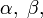$\alpha, \; \beta,$ and$\gamma\;$ are Euler angles (characterized by the keywords: z-y-z convention, right-handed frame, right-hand screw rule, active interpretation).

The Wigner D-matrix is a square matrix of dimension 2j + 1 with general element$D^j_{m'm}(\alpha,\beta,\gamma) \ \stackrel{\mathrm{def}}{=}\ \langle jm' | \mathcal{R}(\alpha,\beta,\gamma)| jm \rangle = e^{-im'\alpha } d^j_{m'm}(\beta)e^{-i m\gamma}.$

The matrix with general element$d^j_{m'm}(\beta)= \langle jm' |e^{-i\beta j_y} | jm \rangle$

is known as Wigner's (small) d-matrix.

###  Wigner (small) d-matrix

Wigner gave the following expression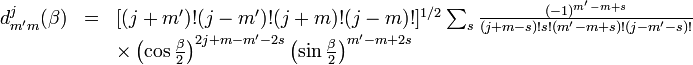$\begin{array}{lcl} d^j_{m'm}(\beta) &=& [(j+m')!(j-m')!(j+m)!(j-m)!]^{1/2} \sum_s \frac{(-1)^{m'-m+s}}{(j+m-s)!s!(m'-m+s)!(j-m'-s)!} \\ &&\times \left(\cos\frac{\beta}{2}\right)^{2j+m-m'-2s}\left(\sin\frac{\beta}{2}\right)^{m'-m+2s} \end{array}$

The sum over s is over such values that the factorials are nonnegative.

Note: The d-matrix elements defined here are real. In the often-used z-x-z convention of Euler angles, the factor ( − 1)m' − m + s in this formula is replaced by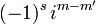$(-1)^s\, i^{m-m'}$, causing half of the functions to be purely imaginary. The realness of the d-matrix elements is one of the reasons that the z-y-z convention, used in this article, is usually preferred in quantum mechanical applications.

The d-matrix elements are related to Jacobi polynomials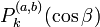$P^{(a,b)}_k(\cos\beta)$ with nonnegative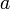$a\,$ and$b\,$.  Let$k = \min(j+m,\,j-m,\,j+m',\,j-m').$$\hbox{If}\quad k = \begin{cases} j+m: &\quad a=m'-m;\quad \lambda=m'-m\\ j-m: &\quad a=m-m';\quad \lambda= 0 \\ j+m': &\quad a=m-m';\quad \lambda= 0 \\ j-m': &\quad a=m'-m;\quad \lambda=m'-m \\ \end{cases}$

Then, with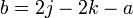$b=2j-2k-a\,$, the relation is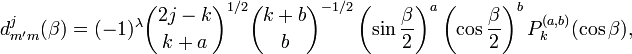$d^j_{m'm}(\beta) = (-1)^{\lambda} \binom{2j-k}{k+a}^{1/2} \binom{k+b}{b}^{-1/2} \left(\sin\frac{\beta}{2}\right)^a \left(\cos\frac{\beta}{2}\right)^b P^{(a,b)}_k(\cos\beta),$

where$a,b \ge 0. \,$

###  Properties of Wigner D-matrix

The complex conjugate of the D-matrix satisfies a number of differential properties that can be formulated concisely by introducing the following operators with$(x,\, y,\,z) = (1,\,2,\,3)$,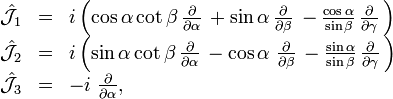$\begin{array}{lcl} \hat{\mathcal{J}}_1 &=& i \left( \cos \alpha \cot \beta \, {\partial \over \partial \alpha} \, + \sin \alpha \, {\partial \over \partial \beta} \, - {\cos \alpha \over \sin \beta} \, {\partial \over \partial \gamma} \, \right) \\ \hat{\mathcal{J}}_2 &=& i \left( \sin \alpha \cot \beta \, {\partial \over \partial \alpha} \, - \cos \alpha \; {\partial \over \partial \beta } \, - {\sin \alpha \over \sin \beta} \, {\partial \over \partial \gamma } \, \right) \\ \hat{\mathcal{J}}_3 &=& - i \; {\partial \over \partial \alpha} , \end{array}$

which have quantum mechanical meaning: they are space-fixed rigid rotor angular momentum operators.

Further,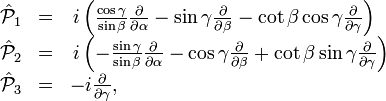$\begin{array}{lcl} \hat{\mathcal{P}}_1 &=& \, i \left( {\cos \gamma \over \sin \beta} {\partial \over \partial \alpha } - \sin \gamma {\partial \over \partial \beta } - \cot \beta \cos \gamma {\partial \over \partial \gamma} \right) \\ \hat{\mathcal{P}}_2 &=& \, i \left( - {\sin \gamma \over \sin \beta} {\partial \over \partial \alpha} - \cos \gamma {\partial \over \partial \beta} + \cot \beta \sin \gamma {\partial \over \partial \gamma} \right) \\ \hat{\mathcal{P}}_3 &=& - i {\partial\over \partial \gamma}, \\ \end{array}$

which have quantum mechanical meaning: they are body-fixed rigid rotor angular momentum operators.

The operators satisfy the commutation relations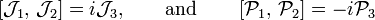$\left[\mathcal{J}_1, \, \mathcal{J}_2\right] = i \mathcal{J}_3, \qquad \hbox{and}\qquad \left[\mathcal{P}_1, \, \mathcal{P}_2\right] = -i \mathcal{P}_3$

and the corresponding relations with the indices permuted cyclically. The$\mathcal{P}_i$ satisfy anomalous commutation relations (have a minus sign on the right hand side).

The two sets mutually commute,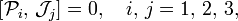$\left[\mathcal{P}_i, \, \mathcal{J}_j\right] = 0,\quad i,\,j = 1,\,2,\,3,$

and the total operators squared are equal,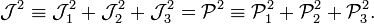$\mathcal{J}^2 \equiv \mathcal{J}_1^2+ \mathcal{J}_2^2 + \mathcal{J}_3^2 = \mathcal{P}^2 \equiv \mathcal{P}_1^2+ \mathcal{P}_2^2 + \mathcal{P}_3^2 .$

Their explicit form is,$\mathcal{J}^2= \mathcal{P}^2 = -\frac{1}{\sin^2\beta} \left( \frac{\partial^2}{\partial \alpha^2} +\frac{\partial^2}{\partial \gamma^2} -2\cos\beta\frac{\partial^2}{\partial\alpha\partial \gamma} \right) -\frac{\partial^2}{\partial \beta^2} -\cot\beta\frac{\partial}{\partial \beta}.$

The operators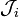$\mathcal{J}_i$ act on the first (row) index of the D-matrix,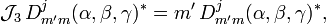$\mathcal{J}_3 \, D^j_{m'm}(\alpha,\beta,\gamma)^* = m' \, D^j_{m'm}(\alpha,\beta,\gamma)^* ,$

and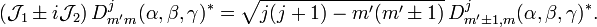$(\mathcal{J}_1 \pm i \mathcal{J}_2)\, D^j_{m'm}(\alpha,\beta,\gamma)^* = \sqrt{j(j+1)-m'(m'\pm 1)} \, D^j_{m'\pm 1, m}(\alpha,\beta,\gamma)^* .$

The operators$\mathcal{P}_i$ act on the second (column) index of the D-matrix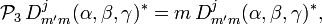$\mathcal{P}_3 \, D^j_{m'm}(\alpha,\beta,\gamma)^* = m \, D^j_{m'm}(\alpha,\beta,\gamma)^* ,$

and because of the anomalous commutation relation the raising/lowering operators are defined with reversed signs,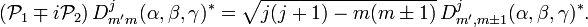$(\mathcal{P}_1 \mp i \mathcal{P}_2)\, D^j_{m'm}(\alpha,\beta,\gamma)^* = \sqrt{j(j+1)-m(m\pm 1)} \, D^j_{m', m\pm1}(\alpha,\beta,\gamma)^* .$

Finally,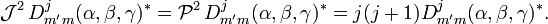$\mathcal{J}^2\, D^j_{m'm}(\alpha,\beta,\gamma)^* = \mathcal{P}^2\, D^j_{m'm}(\alpha,\beta,\gamma)^* = j(j+1) D^j_{m'm}(\alpha,\beta,\gamma)^*.$

In other words, the rows and columns of the (complex conjugate) Wigner D-matrix span irreducible representations of the isomorphic Lie algebra's generated by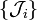$\{\mathcal{J}_i\}$ and$\{-\mathcal{P}_i\}$.

An important property of the Wigner D-matrix follows from the commutation of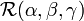$\mathcal{R}(\alpha,\beta,\gamma)$ with the time reversal operator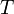$T\,$,$\langle jm' | \mathcal{R}(\alpha,\beta,\gamma)| jm \rangle = \langle jm' | T^{\,\dagger} \mathcal{R}(\alpha,\beta,\gamma) T| jm \rangle = (-1)^{m'-m} \langle j,-m' | \mathcal{R}(\alpha,\beta,\gamma)| j,-m \rangle^*,$

or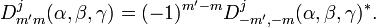$D^j_{m'm}(\alpha,\beta,\gamma) = (-1)^{m'-m} D^j_{-m',-m}(\alpha,\beta,\gamma)^*.$

Here we used that$T\,$ is anti-unitary (hence the complex conjugation after moving$T^\dagger\,$ from ket to bra),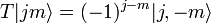$T | jm \rangle = (-1)^{j-m} | j,-m \rangle$  and$(-1)^{2j-m'-m} = (-1)^{m'-m}\,$.

###  Relation with spherical harmonic functions

The D-matrix elements with second index equal to zero, are proportional to spherical harmonics, normalized to unity and with Condon and Shortley phase convention,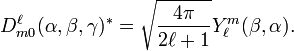$D^{\ell}_{m 0}(\alpha,\beta,\gamma)^* = \sqrt{\frac{4\pi}{2\ell+1}} Y_{\ell}^m (\beta, \alpha ) .$

The left-hand side contains γ in the expression exp[−i⋅0⋅γ] and hence does not depend on γ. The angle γ does not appear in the right-hand side either, so that the expression is valid for any γ. In the present convention of Euler angles, α is a longitudinal angle and β is a colatitudinal angle (spherical polar angles in the physical definition of such angles). This is one of the reasons that the z-y-z convention is used frequently in molecular physics. From the time-reversal property of the Wigner D-matrix follows immediately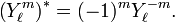$\left( Y_{\ell}^m \right) ^* = (-1)^m Y_{\ell}^{-m}.$